Instant Maths Help:

# Edexcel A Level

## Implicit Differentiation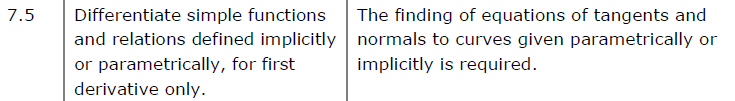##### Edexcel 9MA0/02 Jun 2023 A2 Exam Q. 7 :   7 marks in 8:24 min.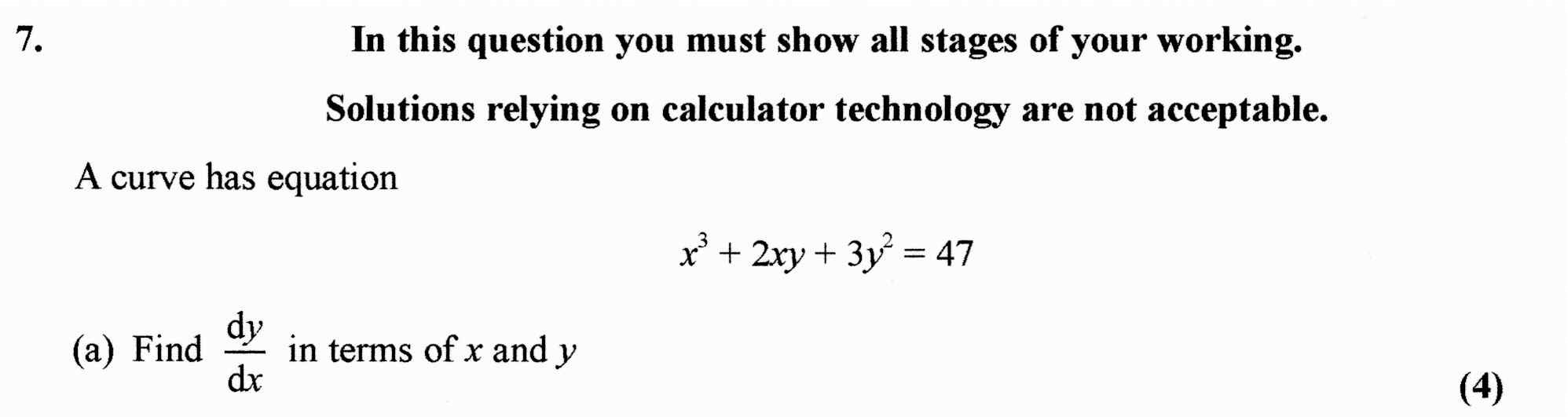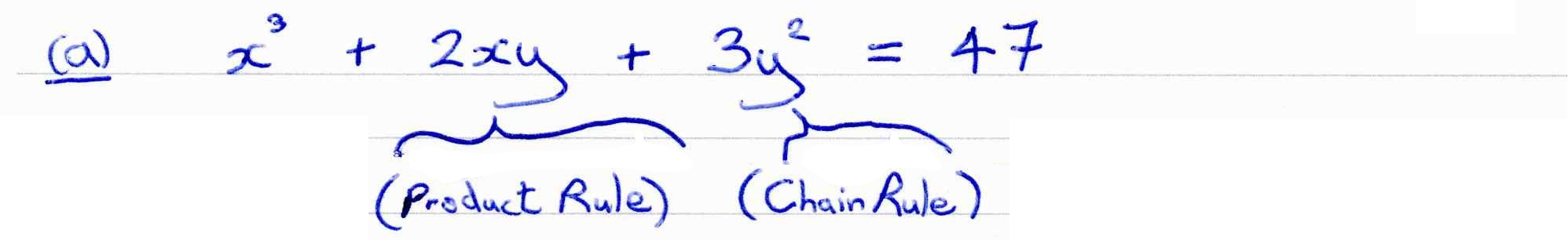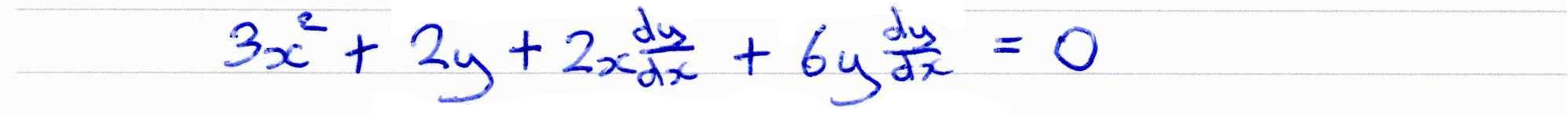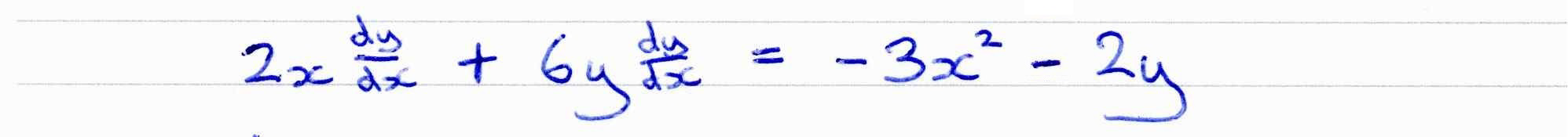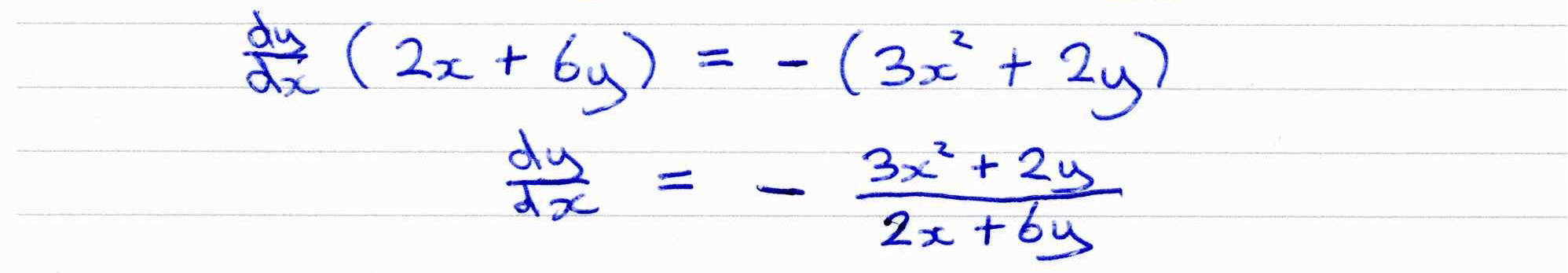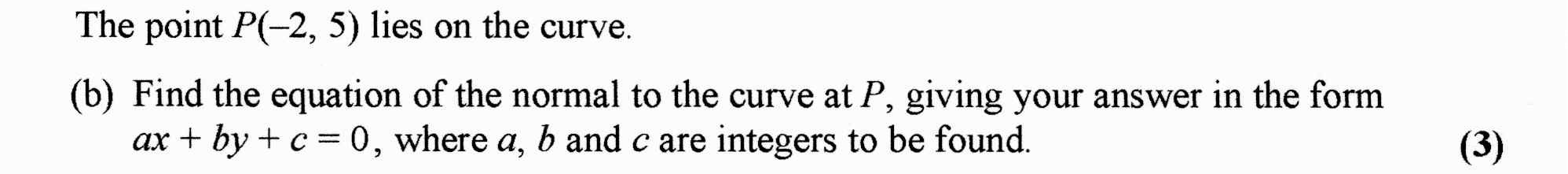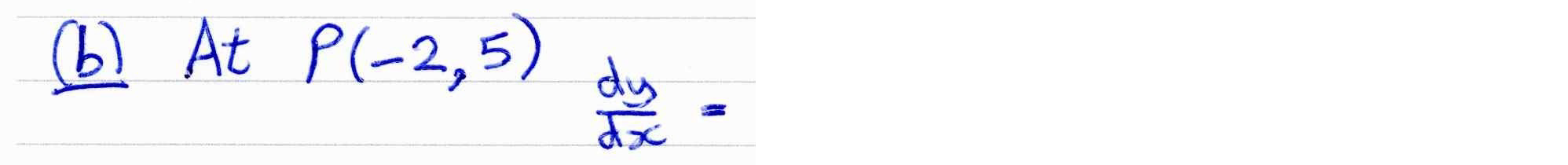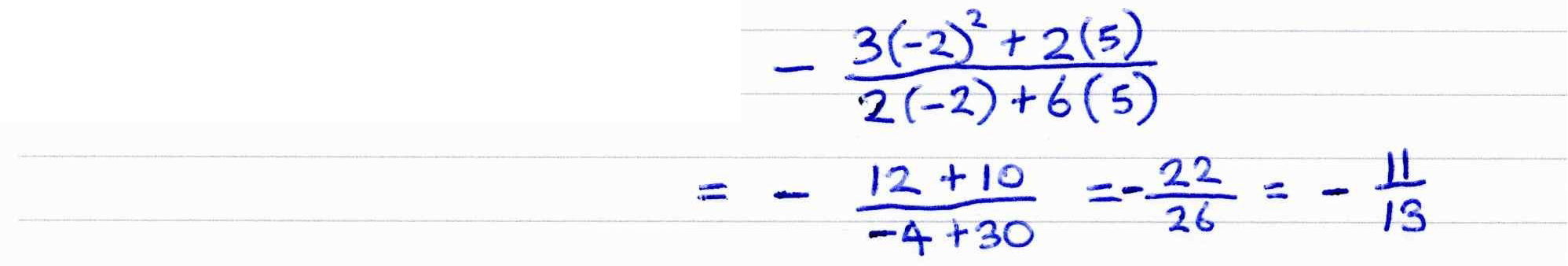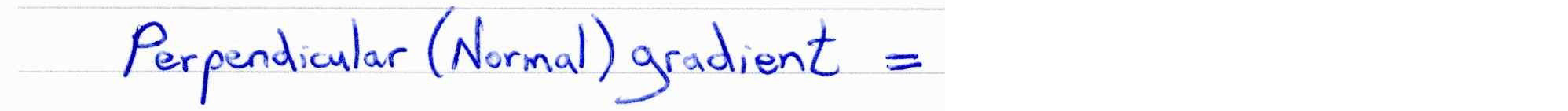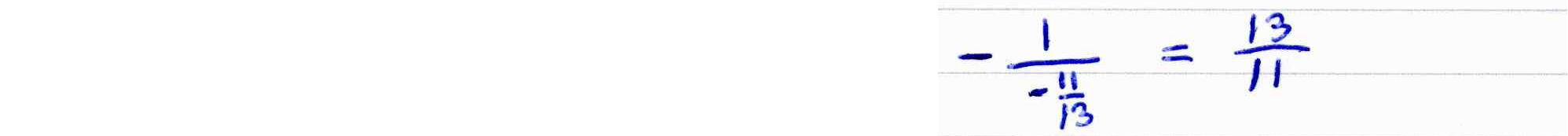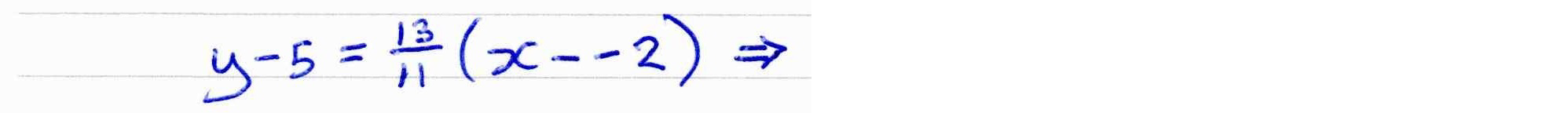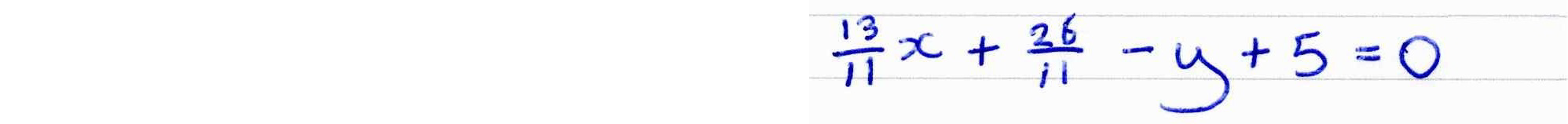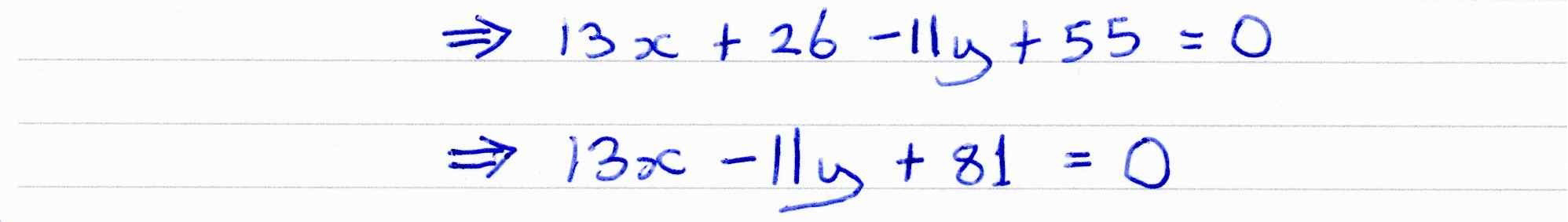##### Chain Rule in Implicit Differentiation
This display shows implicit differentiation of $\color{blue}{y^a = b \, x}$.
Differentiation involves use of the chain rule for $\color{green}{y^a}$.
##### Product/Chain Rules in Implicit Differentiation
This display shows implicit differentiation of $\color{blue}{x^a \, y^b = c}$.
Differentiation involves use of the product rule for $\color{green}{x^a \, y^b}$, and the chain rule for $\color{green}{y^b}$.
##### Implicit Differentiation of Ellipse
A standard cartesian equation of an ellipse is $\color{blue}{{{{x^2}} \over {{a^2}}} + {{{y^2}} \over {{b^2}}} = 1}$,
where a and b are constants.

This display shows implcit differentiation of an ellipse.
##### Implicit Differentiation of Hyperbola
A standard cartesian equation of a hyperbola is $\color{blue}{ { { x^2 } \over { a^2 } } - { { y^2 } \over { b^2 } } = 1 }$,
where a and b are constants.

This display shows implcit differentiation of a hyperbola.